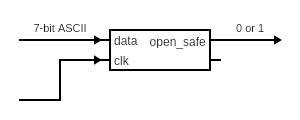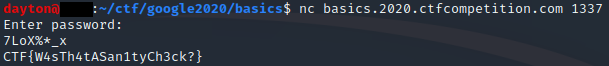30 Aug 2020

# basics

## overview

This challenge was a nice crash course in verilog, which I personally had never worked with. I even had to check to see what type of code was normally contained within a `.sv` file. After reading up on verilog and Verilated, I figured the `main.cpp` file was calling out to the module in `check.sv` and the main goal was to trigger `open_safe`.

## solution

### c++

Before diving into the verilog file, I inspected the code within `main.cpp`:

``````#include "obj_dir/Vcheck.h"

#include <iostream>
#include <memory>

int main(int argc, char *argv[]) {
Verilated::commandArgs(argc, argv);
std::cout << "Enter password:" << std::endl;
auto check = std::make_unique<Vcheck>();

for (int i = 0; i < 100 && !check->open_safe; i++) {
int c = fgetc(stdin);
if (c == '\n' || c < 0) break;
check->data = c & 0x7f;
check->clk = false;
check->eval();
check->clk = true;
check->eval();
}
if (check->open_safe) {
std::cout << "CTF{real flag would be here}" << std::endl;
} else {
std::cout << "=(" << std::endl;
}
return 0;
}
``````

It appeared that I needed to trigger `check->open_safe` to get the flag, which was evaluated within the `for` loop.

The loop will read in whatever is typed through `fgetc()` until either 100 bytes had been entered, the enter key was hit, or if `c < 0`.

Because `c` is AND’d with `0x7f`, which is binary `1111111`, only the last 7 bits of the byte received through `fgetc()` will be used. This means only a password made up of 7-bit ASCII characters will be accepted. At this point, due to only ASCII characters being accepted, I figured this was probably not going to involve much pwning and was probably going to lean more on the reversing side of things.

The following block of code was used to set the clock for `check`:

``````check->clk = false;
check->eval();
check->clk = true;
check->eval();
``````

Because the `check` module triggers its memory storage routine on the clock’s rising edge, it was necessary to bring the clock down and then back up again to move whatever data was in `check->data` into the `check`’s permanent memory.

### verilog

The verilog code contained within `check.sv` seemed to be pretty standard from what I had seen reading up on the language itself:

``````module check(
input clk,

input [6:0] data,
output wire open_safe
);

reg [6:0] memory [7:0];
reg [2:0] idx = 0;

wire [55:0] magic = {
{memory, memory},
{memory, memory},
{memory, memory},
{memory, memory}
};

wire [55:0] kittens = { magic[9:0],  magic[41:22], magic[21:10], magic[55:42] };
assign open_safe = kittens == 56'd3008192072309708;

always_ff @(posedge clk) begin
memory[idx] <= data;
idx <= idx + 5;
end

endmodule
``````

If you are unfamiliar with verilog, it may be easier to think of this code as a component on a circuit board. Verilog is a hardware description language, after all.

The inputs and outputs of this circuit are defined in the following code block:

``````module check(
input clk,

input [6:0] data,
output wire open_safe
);
``````

At a high level, you can think of it like this:At first glance, it looks like we are dealing with the following variables:

• `memory`: an 8-item array of 7-bit items
• `idx`: a 3-bit counter variable, initialized to 0.
• `magic`: a 56-bit ‘wire’ that will contain out of order `memory` items
• `kittens`: a 56-bit ‘wire’ that will contain out of order pieces of data that are going across the `magic` wire

The 7-bit `data` input, which was received from the `for` loop in `main.cpp`, is stored into the 8-item memory array within this block of code:

``````always_ff @(posedge clk) begin
memory[idx] <= data;
idx <= idx + 5;
end
``````

The counter variable `idx` is then incremented by 5.

The goal is to now work backwards.

``````assign open_safe = kittens == 56'd3008192072309708;
``````

This line will send out either true or false (1 or 0) across the output wire `open_safe` if the value stored in `kittens` is equal to the 56-bit decimal number `3008192072309708`.

First, I converted `3008192072309708` into binary since things were being dealt with at the bit-level, which ended up being `00001010101011111110111101001011111000101101101111001100` extended to 56-bits. The next line to inspect would be:

``````wire [55:0] kittens = { magic[9:0],  magic[41:22], magic[21:10], magic[55:42] };
``````

Verilog uses commas within curly brackets as an append operation, so I figured I could split the above binary number into the following:

• `magic[9:0]` = `0000101010`
• `magic[41:22]` = `10111111101111010010`
• `magic[21-10]` = `111110001011`
• `magic[55-42]` = `01101111001100`

I could now assemble what the value is supposed to be within `magic` by putting these numbers together in the correct order, with `magic[55-42]` being the first value: `01101111001100-10111111101111010010-111110001011-0000101010`.

Now, it seemed the last piece of the puzzle was to figure out what was in each `memory` location by seeing how `magic` ordered the values within it:

``````wire [55:0] magic = {
{memory, memory},
{memory, memory},
{memory, memory},
{memory, memory}
};
``````

As stated before, verilog treats comma separted values as one value appended to the other. Each `memory` item is 7-bits in length, so using the value we got for `magic` earlier, the ASCII value of each can be determined:

• `memory` = `0110111` = 7
• `memory` = `1001100` = L
• `memory` = `1011111` = _
• `memory` = `1101111` = o
• `memory` = `0100101` = %
• `memory` = `1111000` = x
• `memory` = `1011000` = X
• `memory` = `0101010` = *

Recall that `idx`, when being used to set the value of `memory[idx]` in the clock positive edge trigger, is incremented by 5. However, it is only a 3-bit value. To account for this, I wrote a simple C program that would increment an 8-bit integer `i` 100 times, mimicking the `for` loop in `main.cpp`, and then proceed to AND it with `111`, or `0x7`:

``````#include <stdio.h>
#include <stdint.h>

int main(int argc, char* argv[]) {
uint8_t i = 0;
// determine order
for(int j = 0; j < 100; j++) {
printf("%d ",i);
i += 5;
i &= 0x7;
}
return 0;
}
``````

This would then spit out all the ordered values of `idx`. The following were the first 8 values produced: `0 5 2 7 4 1 6 3`.

Referring back to the `memory[idx]` to ASCII mappings above, the new order is:

• `memory` = 7
• `memory` = L
• `memory` = o
• `memory` = X
• `memory` = %
• `memory` = *
• `memory` = _
• `memory` = x

Which gives the final password of: `7LoX%*_x`.

Flag: `CTF{W4sTh4tASan1tyCh3ck?}`.